# Quiz 13: Inventory

Business

EOQ analysis is an inventory management technique. It stands for Economic Order Quantity analysis. Carrying costs and ordering costs must be considered in EOQ analysis. Carrying costs refers to the costs of holding the inventories. It is divided into two parts that are written as below: 1. Capital costs: When the company has to pay for the inventory, these costs shows the tied up funds. These funds are used to reduce the borrowed amount. 2. Out-of-pocket costs: These costs includes various expenses like insurance on inventory, loss or damage or theft to inventory, date related issues as expiration of date and so on. Carrying costs are calculated by using the following formula: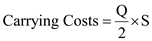Where, Q is the number of units of inventory that is ordered at one time and S is the cost of carrying one unit. Ordering costs refers to the cost of processing the orders related to inventory. Ordering costs are calculated by the following formula: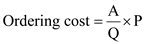Where, A is the total number of units, P is the cost of placing each order and Q is the number of units of inventory that is ordered at one time.

EOQ stands for Economic order quantity which assists the company in determining the most optimum quantity of raw materials which it should hold for production of inventory that it should sell to operate the business. Following the answer obtained from Problem 1, and given the input variables in the question the following graph shall be given as follows: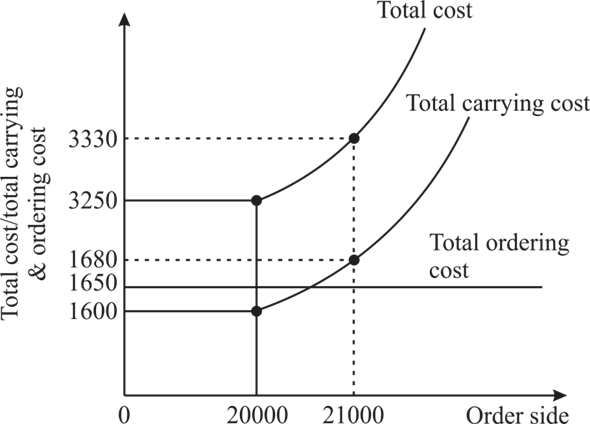In above graph, total carrying cost as well as total cost shall be rising in as per rise in quantity of order. If the quantity was 20,000 units then total cost and total carrying cost is \$3,250 and \$1600 whereas at the time of quantity of 21,000 units the total cost and total carrying cost is \$3,330 and \$1680 respectively. At the EOQ, total cost and total carrying cost shall be equal as the ordering cost shall be minimized and eliminated through determining the EOQ.

The following is the information of Z Hospital: Number of reams of copy paper that Z Hospital purchases each year is 200,000. Price of purchasing each ream of copy paper is \$2.50. Carrying cost of inventory is 6% per annum. Other storing cost of inventory is \$.10 per ream. Ordering cost: Clerical time cost is \$30 and shipping cost is \$135. a. Calculation of Economic Order Quantity (EOQ) is as follows: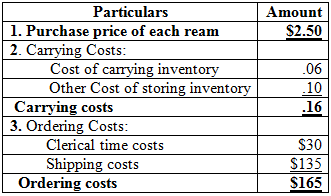In the above table, Carrying costs and ordering costs are calculated. Cost of carrying inventory was 6% per annum that can also be written as.06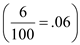. EOQ is calculated by the following formula: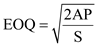Where, A is the total number of units required in one year that is 200,000, P is the cost of placing each order or ordering cost per unit that is \$165 and S is the cost to carry one unit or carrying cost per unit that is \$.16.Therefore, Economic order quantity is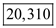units. b. The vendor would deliver the reams in multiples of 1,000 reams of paper, and then the number of reams that should be ordered each time is calculated as below: EOQ is 20,310 units which is calculated in the part-a. As the vendor delivers in multiples of 1,000 reams of paper then 20,000 reams of paper that will have 20 bundles and 21,000 reams of paper that will have 21 bundles would be closest number of bundles that can be ordered. But for the minimum inventory cost, total cost for both will be calculated and whichever of the two is less costly would be the optimal result. Total cost is calculated by the following formula: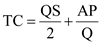Where, A is the total number of units required in one year that is 200,000, P is the cost of placing each order or ordering cost per unit that is \$165, S is the cost to carry one unit or carrying cost per unit that is \$.16 and Q is the number of units that can be ordered in one time that is 20,000 reams of paper or 21,000 reams of paper. For 20,000 reams of paper,For 21,000 reams of paper,Total cost of 20,000 reams of paper is \$3,250 and total cost of 21,000 reams of paper is \$3,330. This shows that 20,000 reams of paper should be ordered each time as it has the less total cost that is \$3,250. Therefore, 20 bundles of reams of paper should be ordered each time and each bundle will contain 1,000 reams of paper. c. The vendor offers a discount of \$.0005 to Z Hospital if it orders 25,000 reams of paper at one time. To know that offer should be accepted or rejected, total costs for 25,000 reams of paper are required to be calculated and is calculated as below:Where, A is the total number of units required in one year that is 200,000, P is the cost of placing each order or ordering cost per unit that is \$165, S is the cost to carry one unit or carrying cost per unit that is \$.16 which will be \$.15 after discount and Q is the number of units that can be ordered in one time that is 25,000 reams of paper.Total cost for ordering 25,000 reams of paper is \$3,195. Total cost at EOQ is calculated as below:Total cost at EOQ is \$3,250. Therefore, total cost of ordering 25,000 reams would be beneficial as its total cost is less than the total cost at EOQ. So, the Z Hospital should accept the offer.